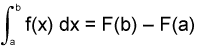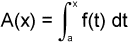# Fundamental Theorem of Calculus

• tdwp

#### tdwp

http://img527.imageshack.us/img527/8089/fr2rl4.gif [Broken]

I know part a is the fundamental theorem of calculus, but I am not quite sure how to manipulate the integral to find part i or part ii.
Part b is again the fundamental theorem of calculus, but I am having a hard time solving for the antiderivative.

Last edited by a moderator:
What is the statement of the fundamental theorem?

Fundamental Theorem of Calculus:

Let f be a function that is continuous on [a,b].
Part 1: Let F be an indefinite integral or antiderivative of f. ThenPart 2:is an indefinite integral or antiderivative of f or A'(x) = f(x)